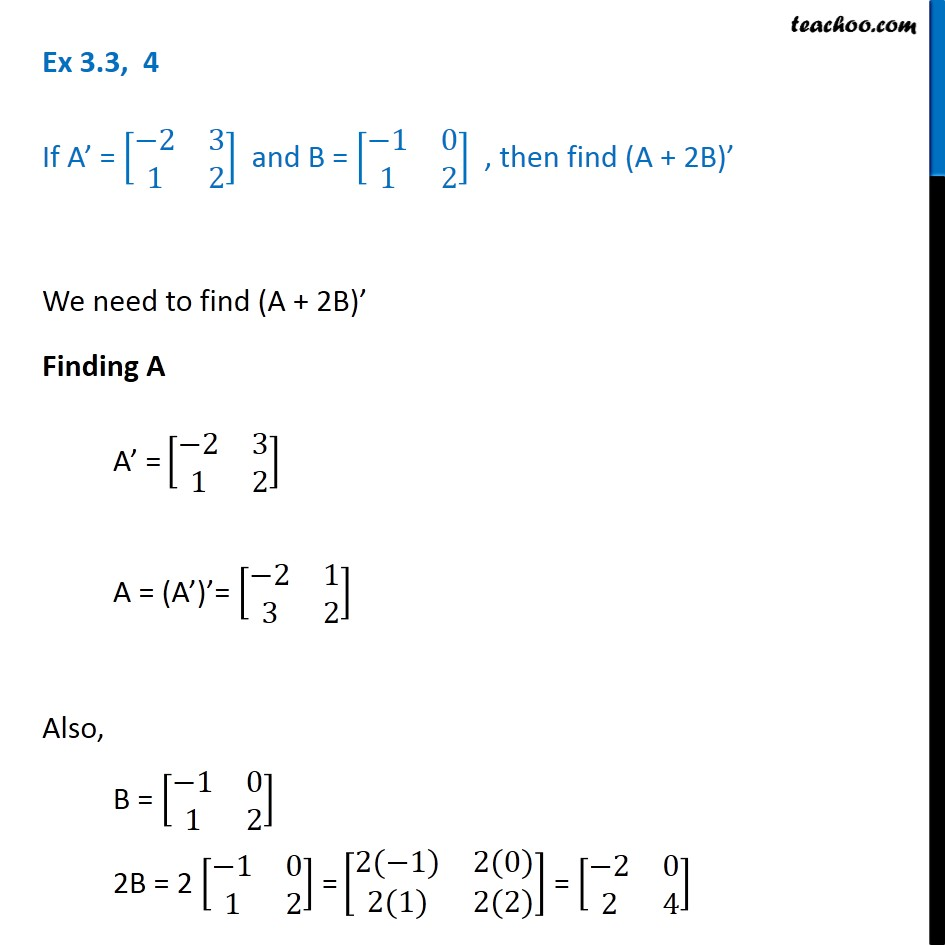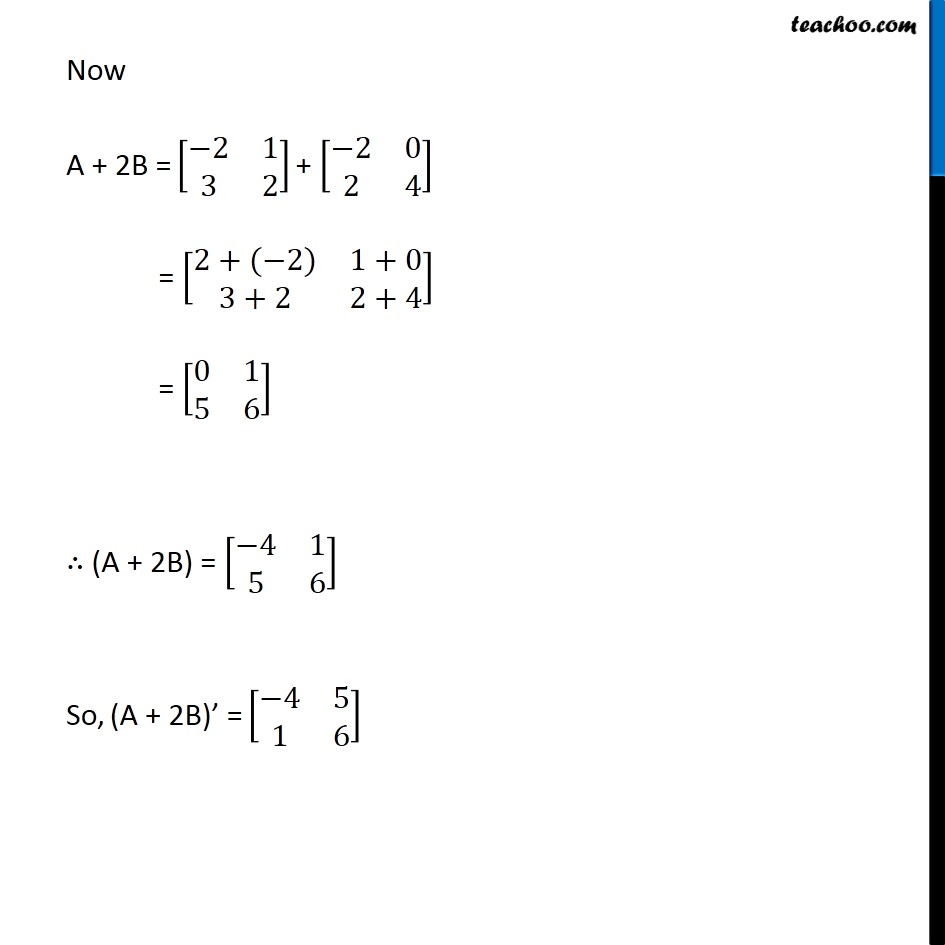Transpose of a matrix

Chapter 3 Class 12 Matrices (Term 1)
Concept wise### Transcript

Ex 3.3, 4 If A’ = [■8(−2&3@1&2)] and B = [■8(−1&0@1&2)] , then find (A + 2B)’ We need to find (A + 2B)’ Finding A A’ = [■8(−2&3@1&2)] A = (A’)’= [■8(−2&1@3&2)] Also, B = [■8(−1&0@1&2)] 2B = 2 [■8(−1&0@1&2)] = [■8(2(−1)&2(0)@2(1)&2(2))] = [■8(−2&0@2&4)] Now A + 2B = [■8(−2&1@3&2)] + [■8(−2&0@2&4)] = [■8(2+(−2)&1+0@3+2&2+4)] = [■8(0&1@5&6)] ∴ (A + 2B) = [■8(−4&1@5&6)] So, (A + 2B)’ = [■8(−4&5@1&6)]